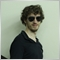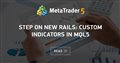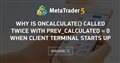# (SOLVED) OnCalculate being called twice with prev_calculated = 01745

Hi!

I'm experiencing an issue I don't recall seeing before: when OnCalculate inside a custom indicator is called for the first time, "prev_calculated" comes with 0. Once the first processing happens, the OnCalculate is finished with "return rates_total". So, in the next call of OnCalculate, prev_calculate = rates_total, that is, the indicator will start the new check from where it stopped before. The exception would be moments where a whole update is called (e.g. by clicking in the Graph with the right button and than in "Update").

Well that is not what is happening to me: I call an indicator, most notably when I open MT5, the indicator is first loaded normally and then, out of nowhere, OnCalculate is once again called with prev_calcualted = 0:

```JN      0       19:40:02.198    teste (WING21,M1)       OnCalculate: rates_total: 10049; prev_calculated: 0
HF      0       19:40:02.199    teste (WING21,M1)       Starting at 100, 2020.10.20 11:41:00; rates_total: 10049
DL      0       19:40:02.199    teste (WING21,M1)       End: rates_total: 10049
EH      0       19:40:02.199    teste (WING21,M1)       OnCalculate: rates_total: 10049; prev_calculated: 10049
HI      0       19:40:02.199    teste (WING21,M1)       End: rates_total: 10049
EO      0       19:40:02.548    teste (WING21,M1)       OnCalculate: rates_total: 10049; prev_calculated: 0
LH      0       19:40:02.548    teste (WING21,M1)       Starting at 100, 2020.10.20 11:41:00; rates_total: 10049
HK      0       19:40:02.548    teste (WING21,M1)       End: rates_total: 10049
```

EA code:

```//+------------------------------------------------------------------+
//|                                  MW teste.mq5 |
//+------------------------------------------------------------------+
#property version "1.0"
//#property description "teste"
//#property icon "Logo-1-Small.ico";

//---- indicator settings
#property indicator_chart_window
#property indicator_buffers 0
#property indicator_plots   0

//--- defines

//--- includes

//--- resources

//--- enums and structs

//--- input parameters

//--- indicator buffers

//--- others

//+------------------------------------------------------------------+
//| Custom indicator initialization function                         |
//+------------------------------------------------------------------+
int OnInit()
{

//---
return INIT_SUCCEEDED;
}
//+------------------------------------------------------------------+
void OnDeinit(const int reason)
{

}
//+------------------------------------------------------------------+
//| Custom indicator iteration function                              |
//+------------------------------------------------------------------+
int OnCalculate(const int rates_total,
const int prev_calculated,
const datetime &time[],
const double &open[],
const double &high[],
const double &low[],
const double &close[],
const long &tick_volume[],
const long &volume[],
{
Print("OnCalculate: rates_total: ",rates_total,"; prev_calculated: ",prev_calculated);

int startPos;
bool isNewCandleRT = false;

const bool isCalculatingForNow = prev_calculated == rates_total;

if (prev_calculated == 0)
{
startPos = 100;

Print("Starting at ",startPos,", ",time[startPos],"; rates_total: ",rates_total);
}
else if (isCalculatingForNow)
{
//Print("Is now");
startPos = MathMax(prev_calculated - 1,1);
}
else
{
//Print("New candle produced");
startPos = prev_calculated;
isNewCandleRT = true;
}

//
for (int aaa = startPos; aaa < rates_total && !IsStopped(); ++aaa)
{

}

//--- return value of prev_calculated for next call
Print("End: rates_total: ",rates_total);
return rates_total;
}```

So why is that second call with prev_calculated = 0 happening? Note: it does so both when the market is open as well as when it's closed.Step on New Rails: Custom Indicators in MQL5
• www.mql5.com
I will not list all of the new possibilities and features of the new terminal and language. They are numerous, and some novelties are worth the discussion in a separate article. Also there is no code here, written with object-oriented programming, it is a too serous topic to be simply mentioned in a context as additional advantages for developers. In this article we will consider the indicators, their structure, drawing, types and their programming details, as compared to MQL4. I hope that this article will be useful both for beginners and experienced developers, maybe some of them will find something new.1745

Martin Bittencourt:

Hi!

I'm experiencing an issue I don't recall seeing before: when OnCalculate inside a custom indicator is called for the first time, "prev_calculated" comes with 0. Once the first processing happens, the OnCalculate is finished with "return rates_total". So, in the next call of OnCalculate, prev_calculate = rates_total, that is, the indicator will start the new check from where it stopped before. The exception would be moments where a whole update is called (e.g. by clicking in the Graph with the right button and than in "Update").

Well that is not what is happening to me: I call an indicator, most notably when I open MT5, the indicator is first loaded normally and then, out of nowhere, OnCalculate is once again called with prev_calcualted = 0:

EA code:

So why is that second call with prev_calculated = 0 happening? Note: it does so both when the market is open as well as when it's closed.

Well I guess I found my answer:

https://www.mql5.com/en/forum/326514

:3Why is Oncalculate() called twice with prev_calculated = 0 when client terminal starts up
• 2019.11.15
• www.mql5.com
If you close the client terminal with a chart and indicator open and then re-open the client terminal, the chart with indicator will appear as expe...24287

Don't try to use any price or server related functions in OnInit (or on load), as there may be no connection/chart yet:

1. Terminal starts.
2. Indicators/EAs are loaded. Static and globally declared variables are initialized. (Do not depend on a specific order.)
3. OnInit is called.
4. For indicators OnCalculate is called with any existing history.
5. Human may have to enter password, connection to server begins.
6. New history is received, OnCalculate called again.
7. New tick is received, OnCalculate/OnTick is called. Now TickValue, TimeCurrent, account information and prices are valid.